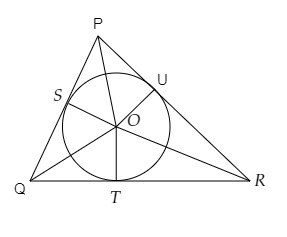# A triangle $P Q R$ is drawn to circumscribe a circle of radius $8 \mathrm{~cm}$ such that the segments $Q T$ and $T R$, into which $Q R$ is divided by the point of contact $T$, are of lengths $14 \mathrm{~cm}$ and $16 \mathrm{~cm}$ respectively. If area of $\Delta P Q R$ is $336 \mathrm{~cm}^{2}$, find the sides $P Q$ and $P R$.

Given:

A triangle $P Q R$ is drawn to circumscribe a circle of radius $8 \mathrm{~cm}$ such that the segments $Q T$ and $T R$, into which $Q R$ is divided by the point of contact $T$, are of lengths $14 \mathrm{~cm}$ and $16 \mathrm{~cm}$ respectively.

Area of $\Delta P Q R$ is $336 \mathrm{~cm}^{2}$.

To do:
We have to find the sides $P Q$ and $P R$.
Solution:$\triangle PQR$ is circumscribed by a circle with centre $O$ and radius $8\ cm$.

$T$ is the point of contact that divides the line segment $OT$ into two parts such that

$QT = 14\ cm$ and $TR = 16\ cm$.

Area of $\triangle PQR = 336\ cm^2$

Let $PS = x\ cm$

$QT$ and $QS$ are tangents to the circle from $Q$.

$QS = QT = 14\ cm$

Similarly,

$RU$ and $RT$ are tangents to the circle

$RT = RU = 16\ cm$

$PS$ and $PU$ are tangents from $P$

$PS = PU = x\ cm$

$PQ = x + 14$ and $PR = x + 16$ and $QR = 14 + 16 = 30\ cm$

Area of $\triangle PQR =$ Area of $\triangle POQ +$ Area of $\triangle QOR +$ Area of $\triangle POR$

$\Rightarrow 336=\frac{1}{2}(\mathrm{QR}) \times 8+\frac{1}{2}(14+x) \times 8+\frac{1}{2}(16+x) \times 8$

$\Rightarrow 336=\frac{1}{2} \times 30 \times 8+4(14+x)+4(16+x)$

$\Rightarrow 336=120+56+4 x+64+4 x$

$\Rightarrow 336=8 x+240$

$\Rightarrow 8 x=336-240$

$\Rightarrow 8x=96$

$\Rightarrow x=\frac{96}{8}=12$

Therefore, $\mathrm{PQ}=x+14=12+14=26 \mathrm{~cm}$

$\mathrm{PR}=x+16=12+16=28 \mathrm{~cm}$

The sides $P Q$ and $P R$ are $26\ cm$ and $28\ cm$ respectively.

Updated on: 10-Oct-2022

33 Views